Latest Banking jobs   »

# Quantitative Aptitude Quiz For IDBI AM/Executive 2022- 22nd June

There are three sitting halls, three pantry, three meeting rooms and one HR & one CEO room in the office of Adda247. All halls, pantry, meeting rooms, HR room & CEO rooms are rectangular in shape and area of each hall is same, each pantry is same, each meeting room is same and area is different for HR & CEO room. Breadth of hall & pantry are 33 m & 15 m respectively, while ratio of length of hall to that of pantry is 22 : 7. The difference between perimeter of a hall & a pantry is 126 m, while area of a meeting room having breadth of 14 m is 77 m² less than that of area of a pantry room. Ratio of magnitude of perimeter of HR room to area of that room is 19 : 88 and breadth of that room is 16 m. Length & breadth of CEO room is 4 m & 2 m more than that of length and breadth of HR room respectively. (Consider only 2D figure)

Quantitative Quiz For IDBI AM/Executive 2022 22nd June CHECK IN HINDI

Q1. The radius of a cylindrical vessel is equal to half of the length of a pantry. Its volume is 4158 m^3 and cost of polishing it is Rs. 5 per m², then find the total cost of polishing the vessel including its top and bottom surfaces.
(a) 7225 Rs.
(b) 7425 Rs.
(c) 7050 Rs.
(d) 7825 Rs.
(e) 7625 Rs.

Q2. The ratio of radius of two circular parks is 5: 6 and sum of circumference of both circular parks is 154m more than perimeter of a CEO room. If cost of fencing per meter with wire is Rs. 25, then find the total cost of fencing the smaller circular park?
(a) 2560 Rs.
(b) 2456 Rs.
(c) 2226 Rs.
(d) 2288 Rs.
(e) 2750 Rs.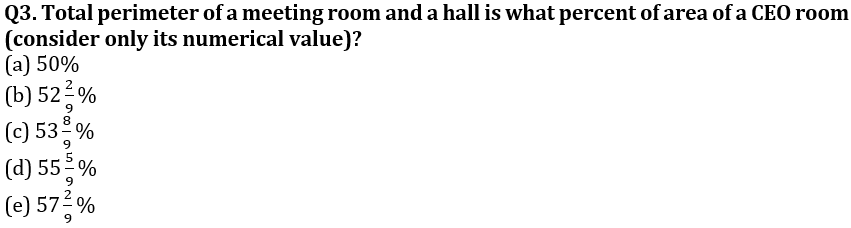Q4. All three meeting rooms are rebuilt so that breadth of each meeting room is increased by 100% and length is decreased by 10 m. If each meeting room is divided into four square cabins and decorated with wood which costs Rs 12.5 per/m², then find the total cost of decoration of all square cabins built in the three meeting rooms ?
(a) 7290 Rs.
(b) 7230 Rs.
(c) 7240 Rs.
(d) 7350 Rs.
(e) 7560 Rs.

Q5. Find the difference between perimeter of a HR room & perimeter of a pantry?
(a) 4 m
(b) 8 m
(c) 12 m
(d) 16 m
(e) 18 m

Directions (6-10): Bar- graph given below shows the selling price(in rupees) of a single item of five products by a shopkeeper and also the profit percent of a single item of each product is given.
Study the graph carefully and answer the question given below.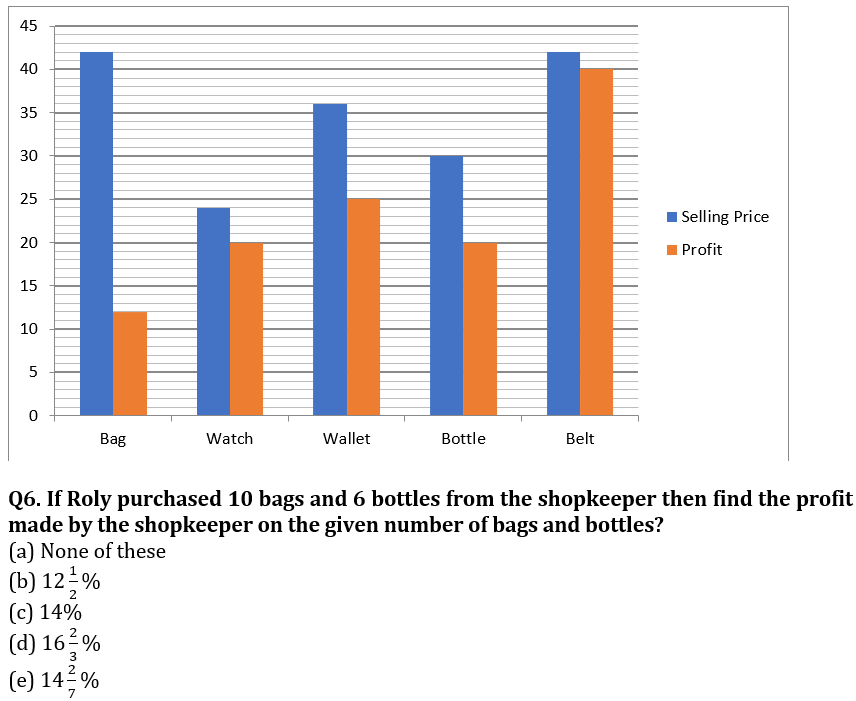Q7. If Abhi purchased 4 wallets and sold two of them at 25% loss and rest at 12.5%profit. Then find the net profit/loss obtained by him.
(a) Rs. 14
(b) Rs. 9
(c) Rs. 6
(d) Rs. 12
(e) none of these

Q8. What is the average of cost price of all five items if single item is considered?
(a) 28.26
(b) 23.25
(c) 32.28
(d) 18
(e) None of these

Q9. If shopkeeper also wants to sell 5 tiffin, the cost price of single tiffin is same as cost price of single watch and made a total profit of 50%. Then find the average of selling price of single tiffin and cost price of single bottle?
(a) 27.5
(b) None of these
(c) 22.5
(d) 18
(e) 15

Q10. If Rahul and Prabhat made profit by selling 5 belts each whose ratio of selling price is 3 : 2 and cost price of one belt is Rs. 30. If profit made by both is equal to profit made by shopkeeper on selling 5 bags. Then find the difference of selling price of Rahul and Prabhat?
(a) Rs. 12.9
(b) Rs. 16.4
(c) Rs. 8.06
(d)Rs. 11.09
(e) none of these

Directions (11-15): Table given below shows total products sold by six sellers. Products are of only two types ‘A’ and ‘B’. Some data is missing in table. Students are expected to calculate the missing data according to questions. Study the data carefully and answer the following questions.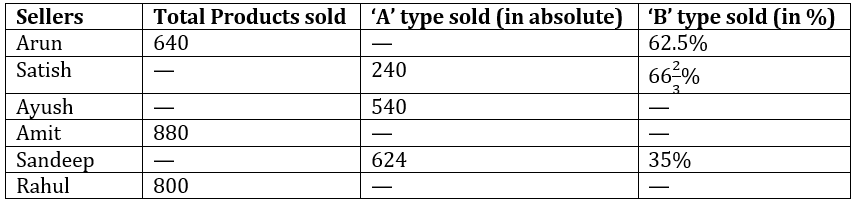Note :- 1. Total Products = ‘A’ type + ‘B’ type

Q11. Total Products sold by Sandeep and Rahul together is what percent more than total products sold by Amit and Satish together?
(a) 5%
(b) 15%
(c) 20%
(d) 10%
(e) 25%

Q12. ‘A’ type product sold by Amit is 37.5% more than ‘A’ type product sold by Satish. Find ‘A’ type product sold by Amit is what percent of ‘B’ type product sold by Amit?
(a) 60%
(b) 50%
(c) 40%
(d)75%
(e)80%

Q13. ‘B’ type product sold by Arun is how much less than ‘B’ type product sold by Ayush if total product sold by Ayush is 87.5% more than total products sold by Arun.
(a) 160
(b) 200
(c) 260
(d) 240
(e) 280

Q14. If ratio of ‘B’ type product sold by Satish to ‘B’ type product sold by Rahul is 4 : 3, then, find the average number of ‘A’ type products sold by Arun and Rahul together?
(a) 360
(b) 310
(c) 320
(d) 330
(e) 340

Q15. If ‘B’ type product sold by Ayush is 25% more than ‘B’ type product sold by Sandeep then, find total product sold by Ayush is what percent more than total product sold by Sandeep?
(a) 0%
(b) 50%
(c) 100%
(d) 150%
(e) 70%

Solutions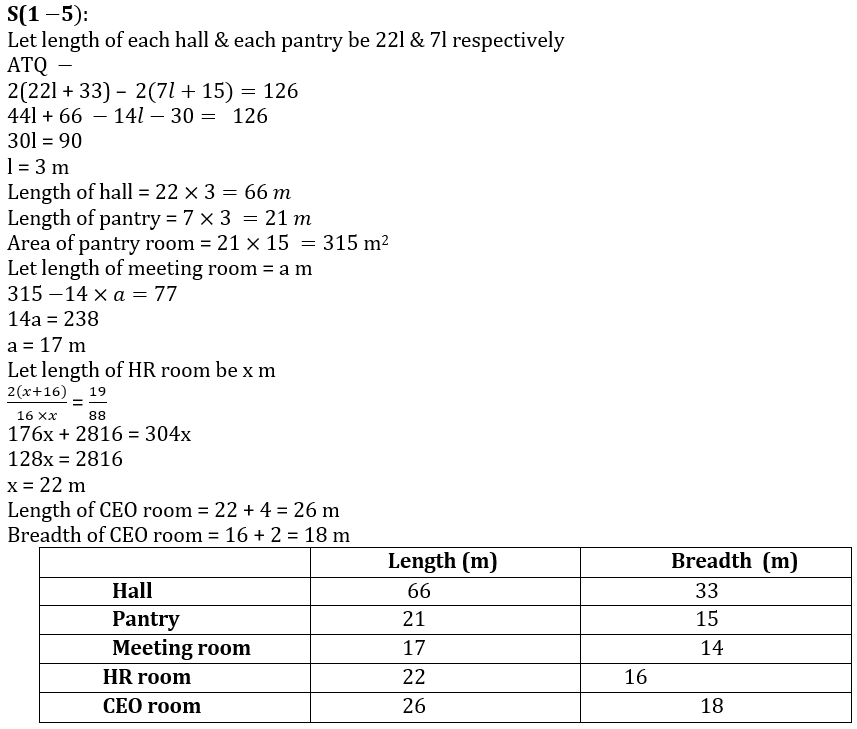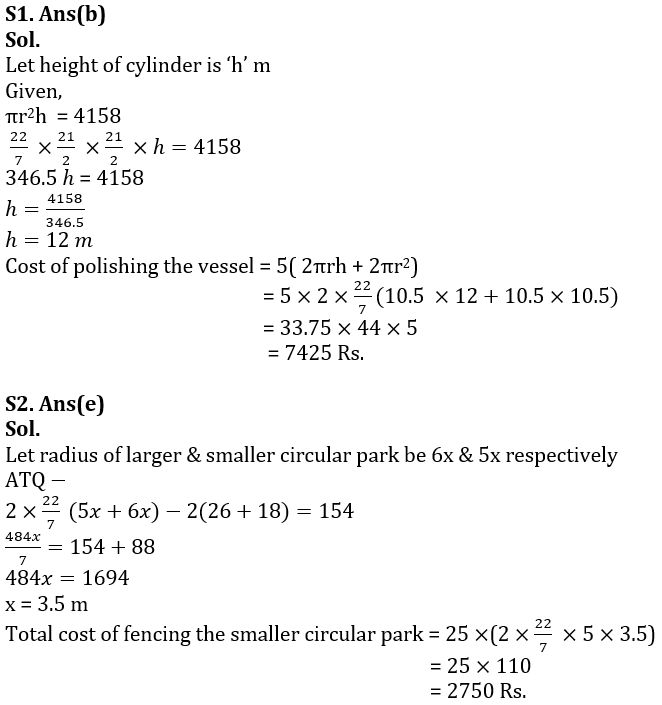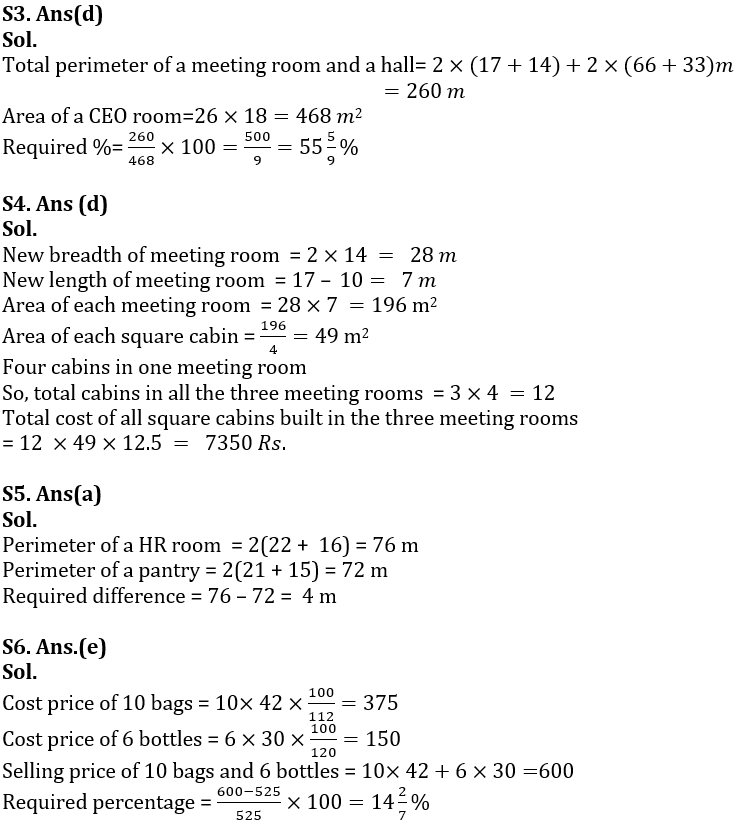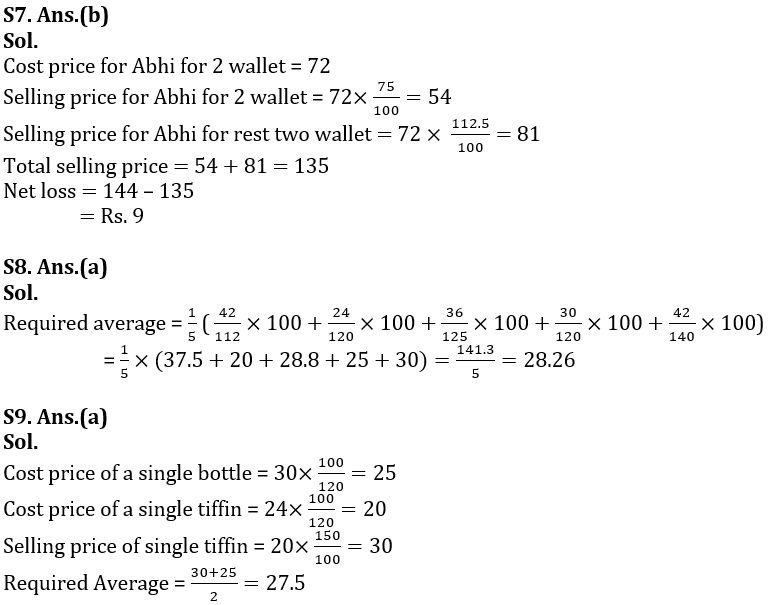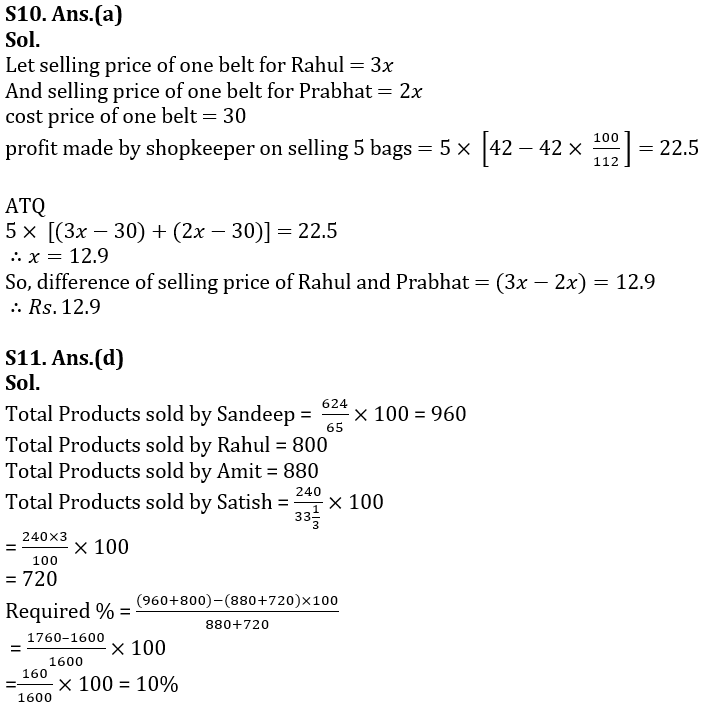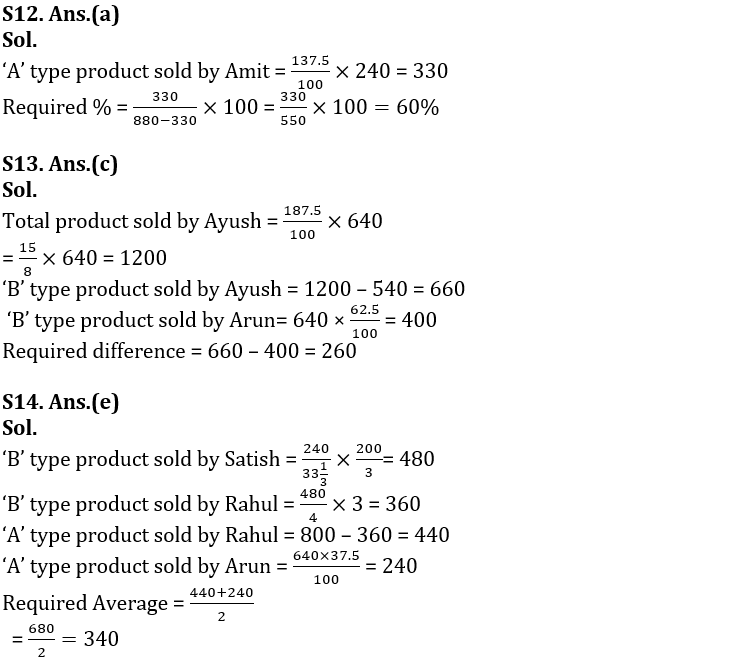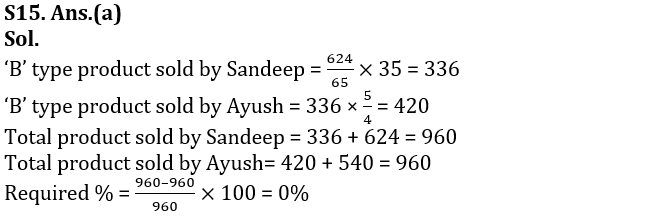#### Congratulations!